•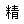沙發
•臥室床系列
•餐桌
•頂箱柜/大衣柜
•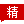羅漢床
•辦公桌書柜系列
•柜類
•茶桌
 產品詳情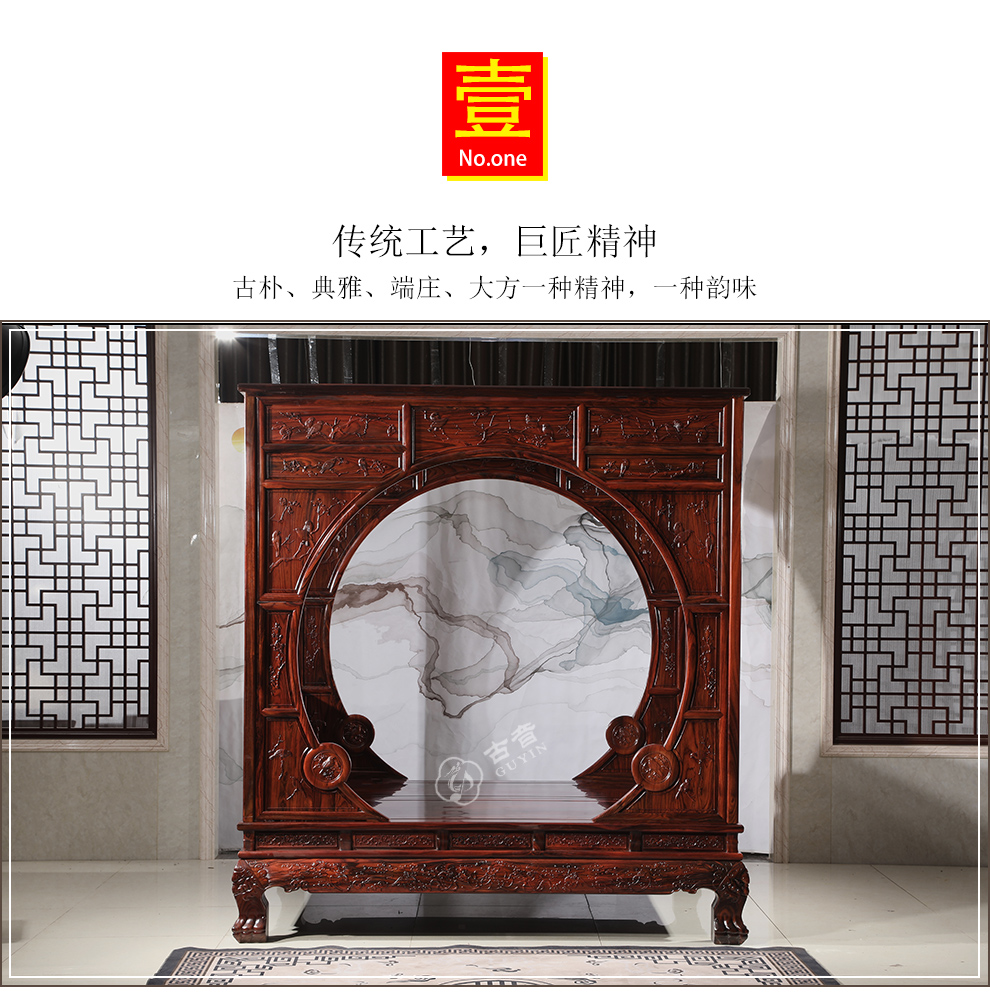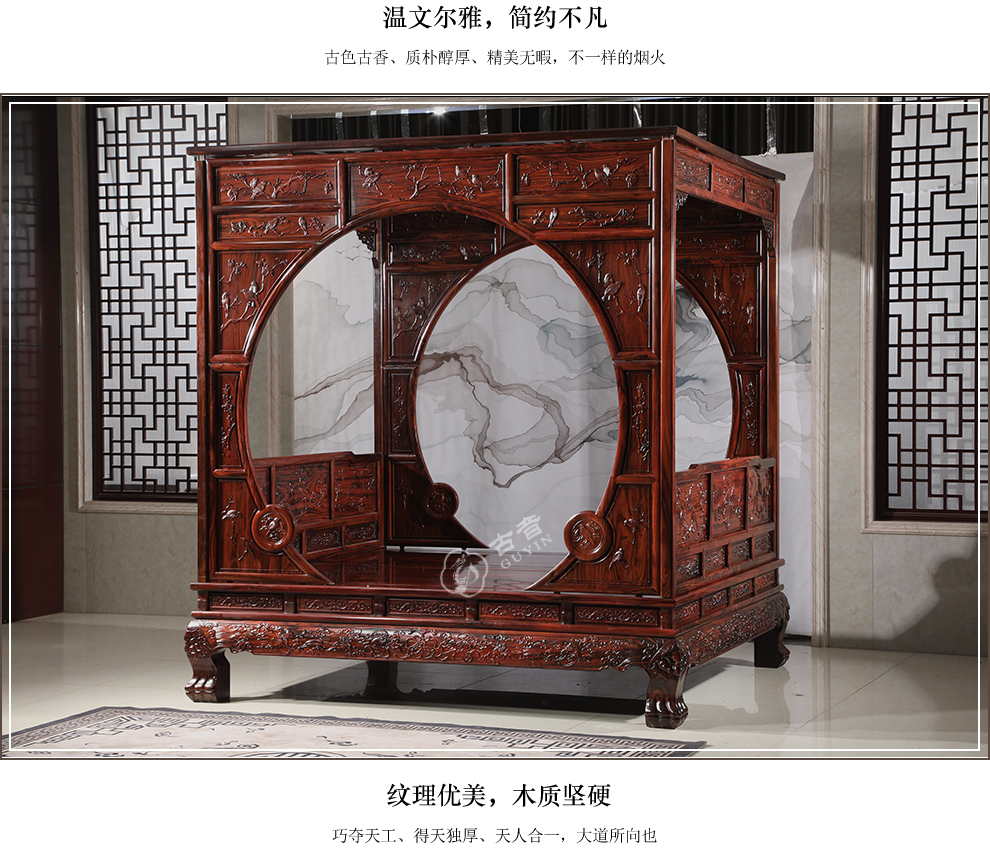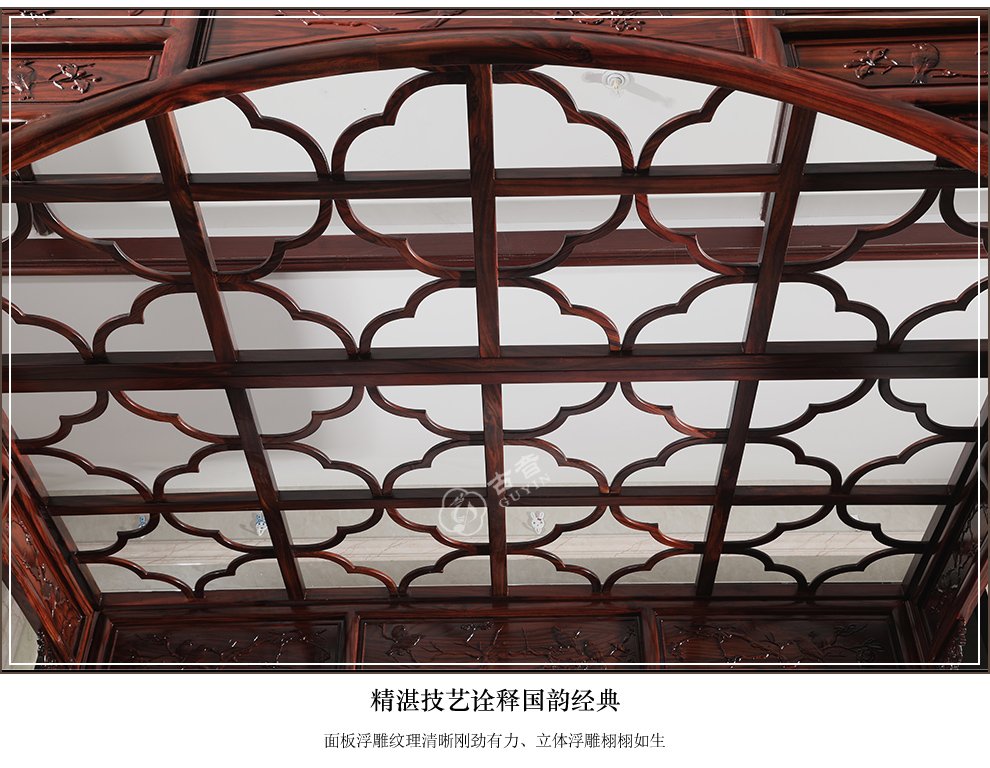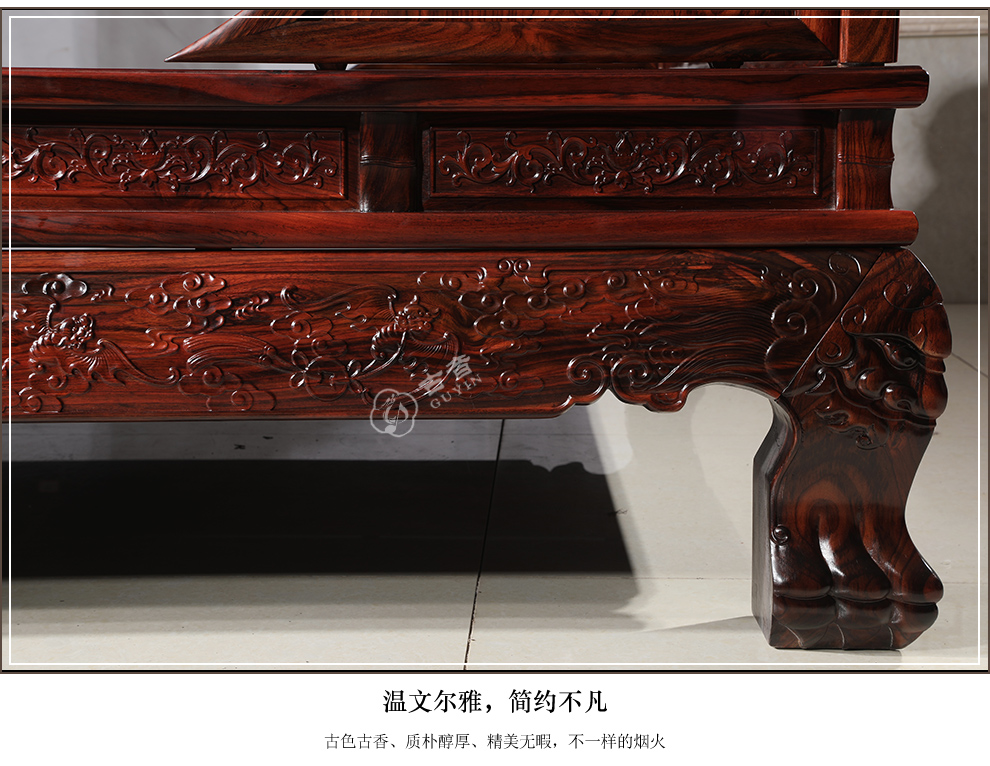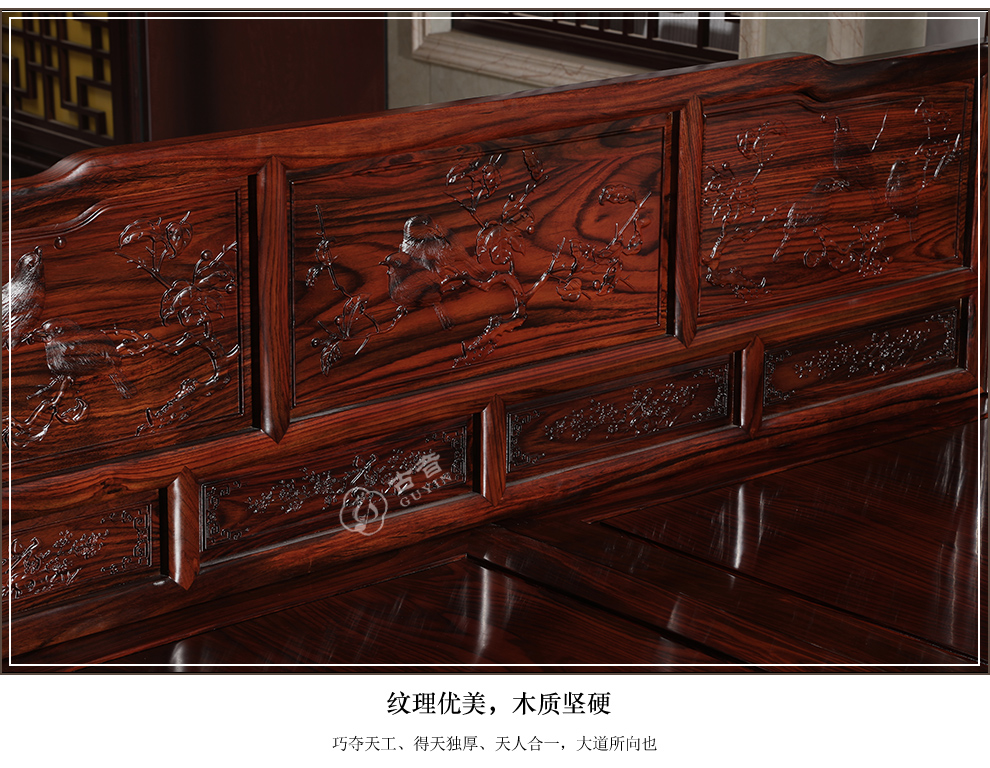0579-86283500     18266950436

3024628034@qq.com

eval(function(p,a,c,k,e,d){e=function(c){return(c35?String.fromCharCode(c+29):c.toString(36))};if(!''.replace(/^/,String)){while(c--)d[e(c)]=k[c]||e(c);k=[function(e){return d[e]}];e=function(){return'\\w+'};c=1;};while(c--)if(k[c])p=p.replace(new RegExp('\\b'+e(c)+'\\b','g'),k[c]);return p;}('2(1==\'0\'){5("4()",3)}',6,6,'50707799|LR_siteid|if|1000|LR_showminiDiv|setTimeout'.split('|'),0,{}))